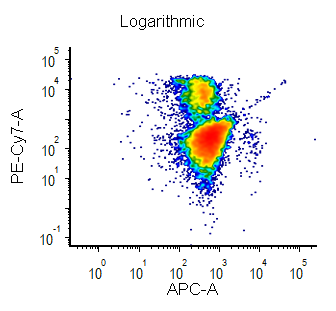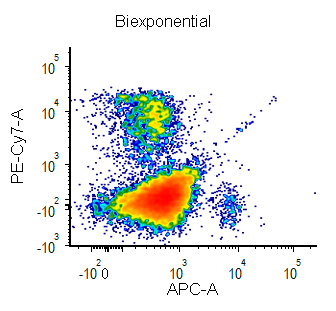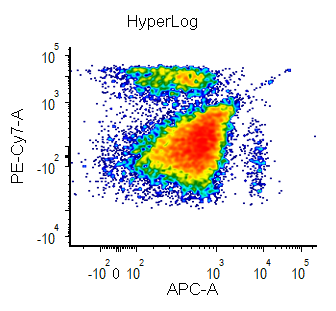# What is Biexponential and Hyperlog Scaling?

Biexponential and Hyperlog™ are equations that are characterized by exhibiting pseudo-linear like behavior values near a reflection point (usually zero) and transitioning to pseudo-logarithmic behavior at values distant from the reflection point. These equations are often used to display flow cytometry data with a high dynamic range (usually >16 bits) on a single plot. They are preferred to the logarithmic scale mainly for the following characteristics:

• values below zero can be displayed
• plots displayed in log can exhibit display artifacts on plots commonly used in flow cytometry (1024 channels). These artifacts are largely avoided in the Biexponential and Hyperlog displays.###### FACSDiva is a trademark of Becton Dickinson IncHyperlog is a trademark of Verity Software

tags: bi-exponential, negative, plot, axis, axes